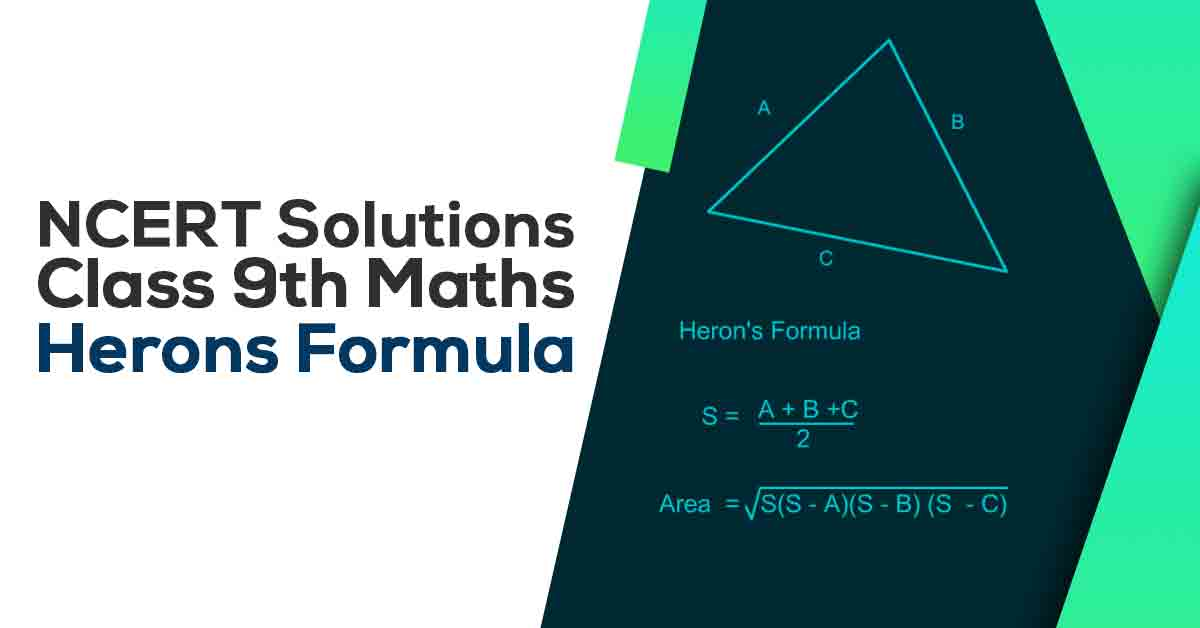NCERT Solutions for Class 9 Maths Chapter 12 | Vidyakul
×

# NCERT Solutions For Class 9 Maths Chapter 12

## NCERT Solutions For Class 9 Maths Chapter 12 Heron’s Formula - PDF DownloadStudents can take help from NCERT Solutions for Class 9 Maths Chapter 12 Heron’s Formula to study for their examinations. In this chapter, we will learn that Area of a quadrilateral whose sides and one diagonal are given, can be calculated by dividing the quadrilateral into two triangles and using the Heron’s formula.

These CBSE NCERT Solutions for Class 9 Maths Chapter 12 Heron’s Formula will help you to practice all the topics in the chapter with ease and score maximum marks in the examination.